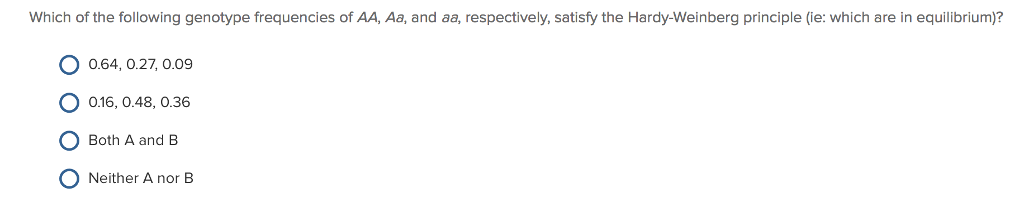# Problem: Which of the following genotype frequencies of AA, Aa, and aa, respectively, satisfy the Hardy-Weinberg principle (i.e. which are in quilibrium)A. 0.64, 0.27, 0.09B. 0.16, 0.48, 0.36C. Both A and BD. Neither E nor B

###### FREE Expert Solution

For a population that follows Hardy-Weinberg equilibrium, the sum of all genotypic frequencies should be equal to 1,###### Problem Details

Which of the following genotype frequencies of AA, Aa, and aa, respectively, satisfy the Hardy-Weinberg principle (i.e. which are in quilibrium)

A. 0.64, 0.27, 0.09

B. 0.16, 0.48, 0.36

C. Both A and B

D. Neither E nor B